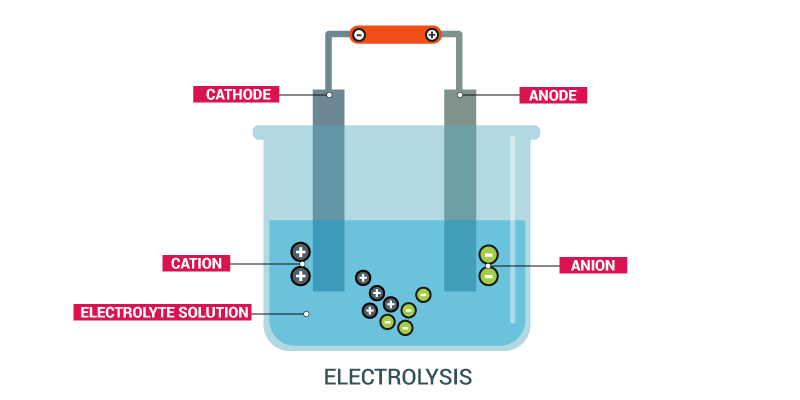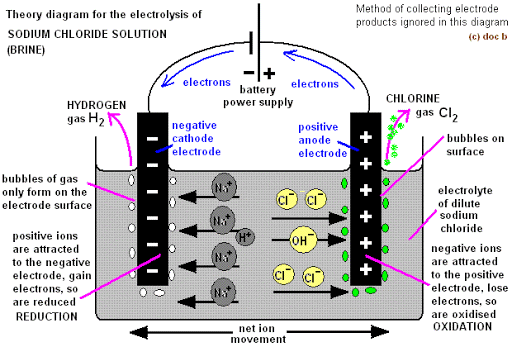# Electrolysis of Specified Electrolytes

Welcome to Class !!

In today’s Chemistry class, We will be learning about the Electrolysis of specified Electrolytes. We hope you enjoy the class!ELECTROLYSIS OF CONCENTRATED SODIUM CHLORIDE SOLUTION (BRINE) USING GRAPHITE ANODE.

Ions present in solution.

NaCl                                                  H2O

Cations                     Na+                                                     H+

Anions                       Cl                                                       OH

At the anode: Both Cl and OH migrate to the anode on passing direct current to compete for discharge. Cl ion being more electronegative and having a high concentration in is discharged in preference to the hydroxide ion, OH, by loss of an electron to produce chlorine atom. Chlorine atoms pair to form chlorine gas.

Anodic half reaction

Cl(aq)              Cl  +e

Cl  +Cl                    Cl2

Overall anodic equation,

2Cl(aq)                      Cl2(g)    +     2e

Two moles of electrons are produced for every mole of chlorine gas formed. These are accepted at the cathode.

At the cathode: Both Na+ and H+ migrate to the cathode on passing direct current to compete for discharge. H+ being far below Na+ and less electropositive is discharged by accepting electrons to produce neutral hydrogen atoms which pair up to produce hydrogen gas. Na+ concentration is high but it is very electropositive and so not discharged.

Cathodic half reaction

H+    +   e                               H

H   +    H                                   H2(g)

Overall cathode reaction

2H+     +      2e                            H2(g)

The overall electrolytic reaction is obtained thus.

2Cl(aq)                            Cl2     +     2e

2H+    +   2e                    H2(g)

2Cl(aq)    +     2H+(aq)                       Cl2(g)   +  H2(g)

The resulting solution becomes more alkaline in NaOH as concentration of Na+ and OH increase.EVALUATION

1. Describe the electrolysis of dilute sodium chloride solution.
2. What is the condition of the final solution?

#### ELECTROLYSIS OF DILUTE H2SO4 (ACIDIFIED WATER)

Acidified water is prepared by adding a few drops of tetraoxosulphate VI acid to water. It is a dilute H2SO4.

Ions present in solution

Compound             H2SO4                         H2O

Cations                     H+                                H+

Anions                       SO42-                           OH

At the anode: Both SO42- and OH migrate to the anode. OH is less electronegative than SO42- and is far above it in the series. It becomes preferentially discharged to produce water and oxygen gas as follows:

Anodic half-reaction

OH (aq)                                   OH  +  e

OH   +  OH                            H2O  + O

O + O                                  O2(g)

Overall anodic reaction

4OH                            2H2O(l)  +   O2   +    4e

At the cathode: H+ is the only cation. The ions migrate to the cathode to accept an electron each to become neutral hydrogen atoms. The atoms pair up to produce hydrogen gas molecules.

Cathodic half equation

H+    +   e       H

H   +    H                  H2(g)

Overall cathodic half equation: 2H+     +     2e–                                     H2

Overall reaction

4OH                            2H2O(l)   +  O2(g)   +   4ex1

2H++  2e                    H2(g)                                       x2

4OH(aq)                     2H2O(l) +   O2 + 4e

4H++  4e                      2H2(g)

4OH+  4H+                   2H2O(l)  +  2H2(g)  + O2(g)#### ELECTROLYSIS OF COPPER (II) TETRAOXOSULPHATE (VI) SOLUTION (USING DIFFERENT ELECTRODES)

Ions in solution

CuSO4           H2O

Cations         Cu2+               H+

Anions           SO42-               OH

At the Anode (Graphite or Platinum): Both SO42-and OH migrate to the anode to compete for discharge. OH is less electronegative and far above SO42-, it is preferentially discharged to produce water and oxygen.

Anodic half reaction:       OH(aq)                       OH       +       e

OH + OH                H2O(l)    +       O

O + O                  O2(g)

Overall anodic half reaction

4OH(aq)                  2H2O(l) +  O2(g)   +   4e

At the Cathode (Graphite or platinum or copper): Both Cu2+ and H+ migrate to the cathode to compete for discharge. Cu2+ is less electropositive and the concentration is high. It becomes discharged as a copper atom.

Cu2+   +    2e-                                 Cu(s)

Overall cell reaction

4OH(aq)                                 2H2O (l)  +  O2 + 4e x1

Cu2+    +   2e                                    Cu(s)               x2

4OH(aq)                                 2H2O (l)  + O2(g)  +   4e

2Cu2+(aq)    +    4e     2Cu(s)

4OH(aq)  +    2Cu2+(aq)                                   2H2O(l)    +      2Cu(s)   +  O2(g)

At the end of the electrolysis, the bluish colour of the electrolyte is lost and the solution becomes more acidic due to the H+ and the SO42- left in the solution.

At the Anode (Copper): Both SO42- and OH migrate to the anode to compete for discharge. Neither SO42- nor OH is discharged but the anode dissolves and goes into the solution as Cu2+.

Cu (s)                                     Cu2+    +    2e

There is no change in the composition of the electrolyte since copper is merely transferred from the anode to the cathode during electrolysis.GENERAL EVALUATION/REVISION

1. State the products formed as the electrode during the electrolysis of CuSO4solution using Cu anode
2. Write balanced half equations for the electrolysis of dilute H2SO4.
3. Describe the electrolysis of dilute sodium chloride solution and state the condition of the final solution.
4. Define the following terms: Atoms, Molecules and Ions.
5. State Dalton’s atomic theory and its modifications.

New School Chemistry for Senior Secondary Schools by O. Y. Ababio, pages 205-207

WEEKEND ASSIGNMENT

SECTION A: Write the correct option ONLY

1. Metal X will be above metal Y in the activity series if X
• A. Displaces ions of Y from solution
• B. Has a higher boiling point than Y
• C. Has a higher melting point than Y
• D. Has a higher relative atomic mass than Y
2. What will happen at the anode when a copper II tetraoxosulphate VI solution is electrolysed using a platinum electrode

A. Copper is dissolved B.         Copper metals is deposited

C. hydrogen is discharged D.        Oxygen gas is produced

3          Oxidation takes place at the anode during electrolysis because the anode

A. Is deficient in electrons     B.         is different in protons

C. has excess electrons            D.     has an attraction for positive ions.

4          What product is formed at the cathode during the electrolysis of concentrated sodium chloride solution using carbon electrode

A. Chlorine        B.         hydrogen     C.        oxygen         D.        sodium

5          Which of the following reactions occurs at the cathode during electrolysis of CuSO4 solution using copper electrodes?

• Cu(s)   –  2e                   Cu2+ (aq)     B.  Cu2+(aq)    –   2e                Cu(s)
• Cu(s)– e                    Cu2+(aq)         D.  Cu2+(aq)   +   2e              Cu(s)

SECTION B

1. State TWO differences between a conductor and an electrolyte.
2. Describe the electrolysis of CuSO4 solution using a platinum electrode.

We have come to the end of this class. We do hope you enjoyed the class?

Should you have any further question, feel free to ask in the comment section below and trust us to respond as soon as possible.

In our next class, we will be learning about Faraday Laws of Electrolysis. We are very much eager to meet you there.

Are you a Parent? Share your quick opinion and win free 2-month Premium Subscription

Don`t copy text!